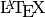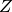Circuit Manipulation and Simulation¶

Introduction¶

Quantum circuits are modeled in QuaEC by a sequence type, qecc.Circuit, that stores zero or more circuit elements, known as locations. Each location has a kind that indicates if it is a gate, measurement or preparation location, as well as which gate, which measurement or which preparation is indicated.

Creating a qecc.Location instance consists of specifying the kind of location along with a sequence of indices indicating which qubits that location acts upon.

>>> import qecc as q
>>> loc = q.Location('CNOT', 0, 2)

The qecc.Location.as_clifford() method allows converting gate locations back into a qecc.Clifford representation if applicable.

>>> print loc.as_clifford()
XII |->  +XIX
IXI |->  +IXI
IIX |->  +IIX
ZII |->  +ZII
IZI |->  +IZI
IIZ |->  +ZIZ

When creating a qecc.Circuit, you may specify each location either as an instance of qecc.Location or as a tuple of arguments to qecc.Location’s constructor.

>>> circ = q.Circuit(('CNOT', 0, 2), ('H', 1), ('X', 0))

Printing a circuit or location results in that instance being represented in the QuASM format, a plaintext representation of quantum circuits.

>>> print loc
CNOT    0 2
>>> print circ
CNOT    0 2
H       1
X       0

The number of qubits, depth and size of each location and circuit can be found by querying the appropriate properties of a qecc.Location or qecc.Circuit:

>>> print loc.nq
3
>>> print circ.nq, circ.depth, circ.size, len(circ)
3 2 3 3

Once constructed, a qecc.Circuit can be transformed in several ways, including simplifications and representations in terms of depth-1 subcircuits.

>>> circ = q.Circuit(('CNOT', 0, 2), ('H', 1), ('X', 0), ('H', 1))
>>> print circ
CNOT    0 2
H       1
X       0
H       1
>>> print circ.cancel_selfinv_gates()
CNOT    0 2
X       0
>>> circ = q.Circuit(('CZ', 0, 2), ('H', 1), ('X', 0))
>>> print circ.replace_cz_by_cnot()
H       2
CNOT    0 2
H       2
H       1
X       0
>>> print "\n    --\n".join(map(str, circ.group_by_time()))
H       2
--
CNOT    0 2
--
H       2
H       1
X       0

Note that, except for qecc.Circuit.group_by_time(), each of these transformations mutates the circuit, so that the original circuit is lost.

>>> print circ
H       2
CNOT    0 2
H       2
H       1
X       0

If a circuit consists entirely of Clifford gate locations, then its entire action may be represented as a qecc.Clifford instance:

>>> circ = q.Circuit(('CZ', 0, 2), ('H', 1), ('X', 0))
>>> print circ.as_clifford()
XII |->  +XIZ
IXI |->  +IZI
IIX |->  -ZIX
ZII |->  -ZII
IZI |->  +IXI
IIZ |->  +IIZ

Finally, circuits can be exported to QCViewer files (*.qcv) for easy integration with QCViewer’s functionality.

>>> print circ.as_qcviewer()
.v q1 q2 q3
.i q1
.o q1
BEGIN
Z    q1 q3
H    q2
X    q1
END

Note that, by default, qubits in the QCViewer export are named “q1”, “q2” and so on. This may be overriden by passing a sequence of strings as the qubit_names argument. Which qubits get assigned to the .i and .o headers in the QCViewer file are controlled by the inputs and outputs arguments, respectively.

>>> print circ.as_qcviewer(inputs=(0,), outputs=(0,), qubit_names=["in1", "anc1", "anc2"])
.v in1 anc1 anc2
.i in1
.o in1
BEGIN
Z    in1 anc2
H    anc1
X    in1
END

qecc.Location: Class representing locations in a circuit¶

Class Reference¶

class qecc.Location(kind, *qubits)[source]

Represents a gate, wait, measurement or preparation location in a circuit.

Note that currently, only gate locations are implemented.

Parameters: kind (int or str) – The kind of location to be created. Each kind is an abbreviation drawn from Location.KIND_NAMES, or is the index in Location.KIND_NAMES corresponding to the desired location kind. qubits (tuple of ints.) – Indicies of the qubits on which this location acts.
KIND_NAMES = ['I', 'X', 'Y', 'Z', 'H', 'R_pi4', 'CNOT', 'CZ', 'SWAP']

Names of the kinds of locations used by QuaEC.

static from_quasm(source)[source]

Returns a qecc.Location initialized from a QuASM-formatted line.

Return type: qecc.Location The location represented by the given QuASM source.
kind

Returns a string defining which kind of location this instance represents. Guaranteed to be a string that is an element of Location.KIND_NAMES.

qubits

Returns a tuple of ints describing which qubits this location acts upon.

nq

Returns the number of qubits in the smallest circuit that can contain this location without relabeling qubits. For a qecc.Location loc, this property is defined as 1 + max(loc.nq).

is_clifford

Returns True if and only if this location represents a gate drawn from the Clifford group.

wt

Returns the number of qubits on which this location acts.

as_clifford(nq=None)[source]

If this location represents a Clifford gate, returns the action of that gate. Otherwise, a RuntimeError is raised.

Parameters: nq (int) – Specifies how many qubits to represent this location as acting upon. If not specified, defaults to the value of the nq property. qecc.Clifford
as_qcviewer(qubit_names=None)[source]

Returns a representation of this location in a format suitable for inclusion in a QCViewer file.

Parameters: qubit_names – If specified, the given aliases will be used for the qubits involved in this location when exporting to QCViewer. Defaults to “q1”, “q2”, etc. str

Note that the identity (or “wait”) location requires the following to be added to QCViewer’s gateLib:

NAME wait
DRAWNAME "1"
SYMBOL I
1 , 0
0 , 1
relabel_qubits(relabel_dict)[source]

Returns a new location related to this one by a relabeling of the qubits. The relabelings are to be indicated by a dictionary that specifies what each qubit index is to be mapped to.

>>> import qecc as q
>>> loc = q.Location('CNOT', 0, 1)
>>> print loc
CNOT    0 1
>>> print loc.relabel_qubits({1: 2})
CNOT    0 2
Parameters: relabel_dict (dict) – If i is a key of relabel_dict, then qubit i will be replaced by relabel_dict[i] in the returned location. qecc.Location A new location with the qubits relabeled as specified by relabel_dict.

qecc.Circuit: Class modeling arrangements of locations¶

Class Reference¶

class qecc.Circuit(*locs)[source]
append(newval)[source]

L.append(object) – append object to end

insert(at, newval)[source]

L.insert(index, object) – insert object before index

nq

Returns the number of qubits on which this circuit acts.

size

Returns the number of locations in this circuit. Note that this property is synonymous with len, in that len(circ) == circ.size for all qecc.Circuit instances.

depth

Returns the minimum number of timesteps required to implement exactly this circuit in parallel.

static from_quasm(source)[source]

Returns a qecc.Circuit object from a QuASM-formatted file, producing one location per line.

as_quasm()[source]

Returns a representation of the circuit in an assmembler-like format. In this format, each location is represented by a single line where the first field indicates the kind of location and the remaining fields indicate the qubits upon which the location acts.

>>> import qecc as q
>>> circ = q.Circuit(('CNOT', 0, 2), ('H', 2), ('SWAP', 1, 2), ('I', 0))
>>> print circ.as_quasm()
CNOT    0 2
H       2
SWAP    1 2
I       0
as_qcviewer(inputs=(0, ), outputs=(0, ), qubit_names=None)[source]

Returns a string representing this circuit in the format recognized by QCViewer.

Parameters: inputs (tuple) – Specifies which qubits should be marked as inputs in the exported QCViewer circuit. outputs (tuple) – Specifies which qubits should be marked as outputs in the exported QCViewer circuit. qubit_names – Names to be used for each qubit when exporting to QCViewer.
as_qcircuit(C=None, R=None)[source]

Typesets this circuit using the Qcircuit package for.

Parameters: C (float) – Width (in ems) of each column. R (float) – Height (in ems) of each column. str A string containingsource code for use with Qcircuit.
as_clifford()[source]

If this circuit is composed entirely of Clifford operators, converts it to a qecc.Clifford instance representing the action of the entire circuit. If the circuit is not entirely Clifford gates, this method raises a RuntimeError.

cancel_selfinv_gates(start_at=0)[source]

Transforms the circuit, removing any self-inverse gates from the circuit if possible. Note that not all self-inverse gates are currently supported by this method.

Parameters: start_at (int) – Specifies which location to consider first. Any locations before start_at are not considered for cancelation by this method.
replace_cz_by_cnot()[source]

Changes all controlled-gates in this circuit to controlled-NOT gates, adding Hadamard locations as required.

Returns an iterator onto subcircuits of this circuit, each of depth 1.

Parameters: pad_with_waits (bool) – If True, each subcircuit will have wait locations added such that every qubit is acted upon in every subcircuit. each depth-1 subcircuit, corresponding to time steps of the circuit

Returns a copy of the qecc.Circuit self, which contains explicit wait locations.

relabel_qubits(relabel_dict)[source]

Returns a new circuit related to this one by a relabeling of the qubits. The relabelings are to be indicated by a dictionary that specifies what each qubit index is to be mapped to.

>>> import qecc as q
>>> loc = q.Location('CNOT', 0, 1)
>>> print loc
CNOT    0 1
>>> print loc.relabel_qubits({1: 2})
CNOT    0 2
Parameters: relabel_dict (dict) – If i is a key of relabel_dict, then qubit i will be replaced by relabel_dict[i] in the returned circuit. qecc.Circuit A new circuit with the qubits relabeled as specified by relabel_dict.

Functions Acting on qecc.Circuit¶

qecc.propagate_fault(circuitlist, fault)[source]

Given a list of circuits representing a list of timesteps (see qecc.Circuit.group_by_time()) and a Pauli fault, propagates that fault through the remainder of the time-sliced circuit.

Parameters: circuitlist (list) – A list of qecc.Circuit instances representing the timesteps of a larger circuit. fault (qecc.Pauli) – A Pauli fault to occur immediately before timestep timestep. timestep (int) – The timestep immediately following when the fault to be propagated occured. qecc.Pauli The effective fault after propagating fault through the remainder of circuitlist.
qecc.possible_faults(circuit)[source]

Takes a sub-circuit which has been padded with waits, and returns an iterator onto Paulis which may occur as faults after this sub-circuit.

Parameters: circuit (qecc.Circuit) – Subcircuit to in which faults are to be considered.
qecc.possible_output_faults(circuitlist)[source]

Gives an iterator onto all possible effective faults due to 1-fault paths occuring within circuitlist, assuming it has been padded with waits.

Parameters: circuitlist (list) – A list of qecc.Circuit instances representing timesteps in a larger circuit. See qecc.Circuit.group_by_time(). qecc.Pauli instances representing possible effective faults due to 1-fault paths within the circuit represented by circuitlist.